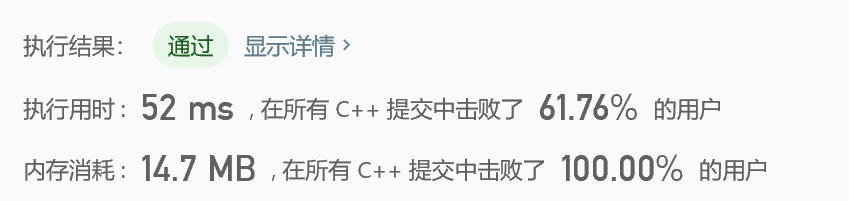# leetcode算法练习——判断子序列

## 题目：

s = "abc", t = "ahbgdc"

s = "axc", t = "ahbgdc"

## 代码如下：

class Solution {
public:
bool isSubsequence(string s, string t)
{
int m(0);
for (int i = 0; i < s.size(); i++)
{
while (m < t.size())
{
if (t[m] == s[i]) break;
m += 1;
}
m += 1;
if (m > t.size()) return false;
}
return true;
}
};

## 运行结果：©️2019 CSDN 皮肤主题: 大白 设计师: CSDN官方博客